# 2nd PUC Maths Question Bank Chapter 9 Differential Equations Ex 9.6

Students can Download Maths Chapter 9 Differential Equations Ex 9.6 Questions and Answers, Notes Pdf, 2nd PUC Maths Question Bank with Answers helps you to revise the complete Karnataka State Board Syllabus and score more marks in your examinations.

## Karnataka 2nd PUC Maths Question Bank Chapter 9 Differential Equations Ex 9.6

### 2nd PUC Maths Differential Equations NCERT Text Book Questions and Answers Ex 9.6

For each of the differential equations given in Exercises 1 to 12, find the general solution:

Question 1.
$$\frac{d y}{d x}+2 y=\sin x$$Question 2.
$$\frac{d y}{d x}+3 y=e^{-2 x}$$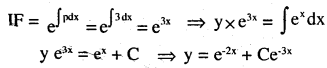Question 3.
$$\frac{d y}{d x}+\frac{y}{x}=x^{2}$$Question 4.
$$\frac{d y}{d x}+(\sec x) y=\tan x\left(0 \leq x<\frac{\pi}{2}\right)$$Question 5.
$$\cos ^{2} x \frac{d y}{d x}+y=\tan x\left(0 \leq x<\frac{\pi}{2}\right)$$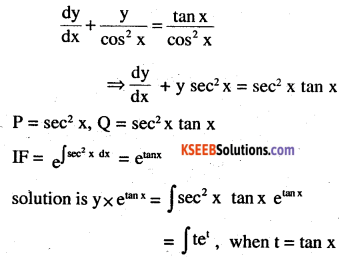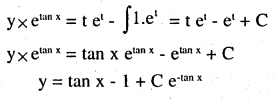Question 6.
$$x \frac{d y}{d x}+2 y=x^{2} \log x$$Question 7.
$$x \log x \frac{d y}{d x}+y=\frac{2}{x} \log x$$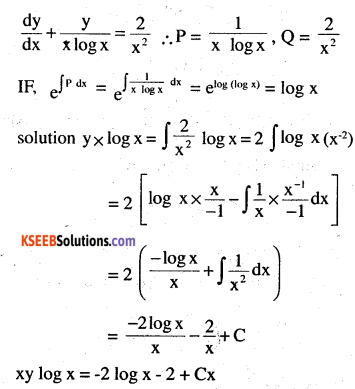Question 8.
(1 + x2) dy + 2xy dx = cot x dx (x≠O)
(1 + x2) dy = (cot x – 2xy) dx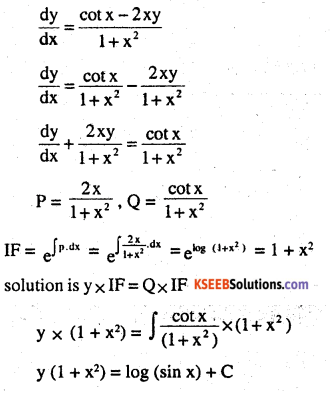Question 9.
$$x \frac{d y}{d x}+y-x+x y \text { cot } x=0(x \neq 0)$$Question 10.
$$(x+y) \frac{d y}{d x}=1$$Question 11.
y dx + (x – y2) dy = 0 .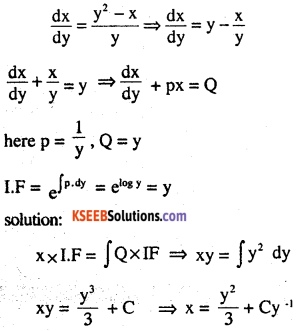Question 12.
$$\left(x+3 y^{2}\right) \frac{d y}{d x}=y(y>0)$$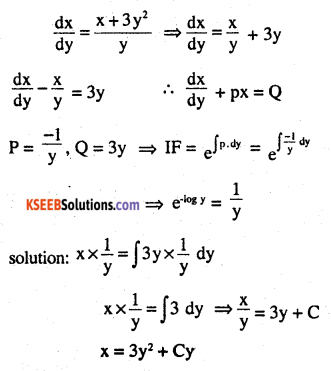For each of the differential equations given in Exercise 13 to 15, find a particular solution satisfying the given condition:

Question 13.
$$\frac{d y}{d x}+2 y \tan x=\sin x ; y=0 \text { when } x=\frac{\pi}{3}$$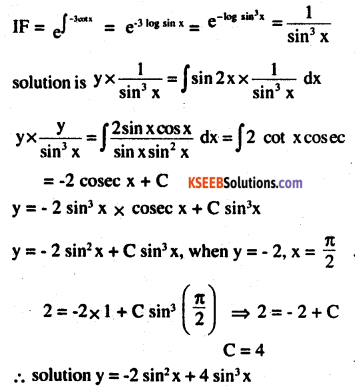Question 14.
$$\left(1+x^{2}\right) \frac{d y}{d x}+2 x y=\frac{1}{1+x^{2}} ; y=0 \text { when } x=1$$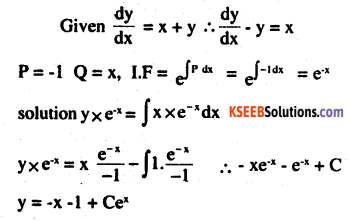Question 15.
$$\frac{d y}{d x}-3 y \cot x=\sin 2 x ; y=2 \text { when } x=\frac{\pi}{2}$$
P = – 3 cot x, Q = sin 2xQuestion 16.
Find the equation of a curve passing through the origin, given that the slope of the tangent to the curve at any point (x, y) is equal to the sum of the coordinates of the point.Question 17.
Find the equation of a curve passing through the point (0,2) given that the sum of the coordinates of any point on the curve exceeds the magnitude of the slope of the tangent to the curve at that point by 5.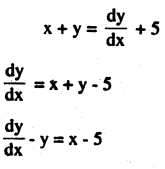Question 18.
The Integrating Factor of the differential equation $$x \frac{d y}{d x}-y=2 x^{2} \text { is }$$
(A) e-x
(B) e-y
(C) $$\frac{1}{x}$$
(D) x$$\left(1-y^{2}\right) \frac{d x}{d y}+y x=a y(-1<y<1) \text { is }$$
(A) $$\frac{1}{y^{2}-1}$$
(B) $$\frac{1}{\sqrt{y^{2}-1}}$$
(C) $$\frac{1}{1-y^{2}}$$
(D) $$\frac{1}{\sqrt{1-y^{2}}}$$